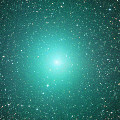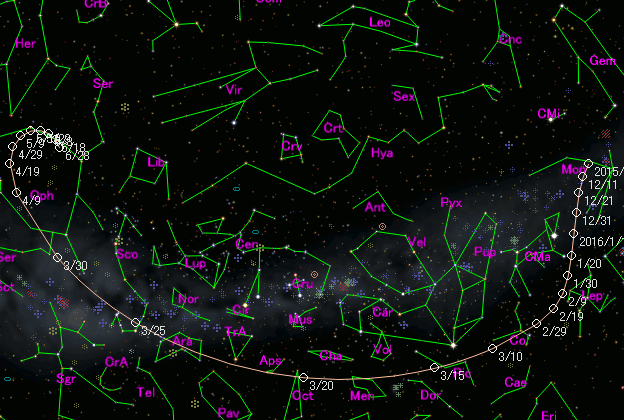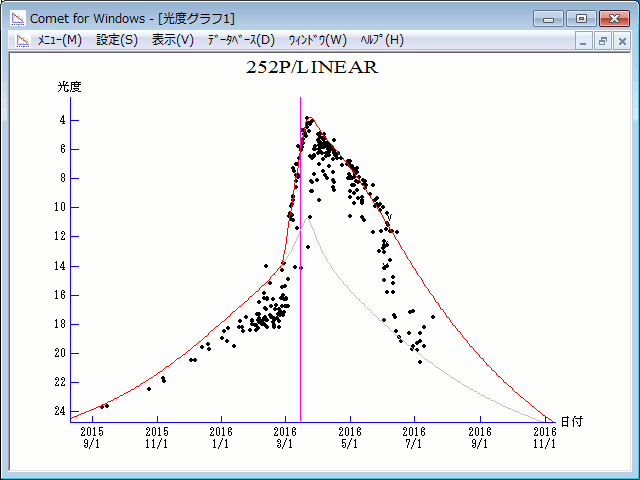252P/LINEAR (2016)PicturesOrbital Elements

Epoch 2016 Apr. 2.0 TT = JDT 2457480.5
T 2016 Mar. 15.27048 TT                                 MPCW
q   0.9960715            (2000.0)            P               Q
n   0.18517038     Peri.  343.31458     -0.99409647     -0.10291243
a   3.0485152      Node   190.95145     +0.10805947     -0.96758658
e   0.6732601      Incl.   10.42289     -0.00976508     -0.23061925
P   5.32
From 1382 observations 2011 June 9-2016 July 31, mean residual 0".8.
Nongravitational parameters A1 = +0.73, A2 = -0.1285.Finding ChartsMagnitudes Graph

m1 = 18.0 + 5 log d +  10.0 log r          [   ,-17]  (             - 2016 Feb. 27)
m1 = 10.5 + 5 log d + 170   log r(t - 18)  [-17, 16]  (2016 Feb. 27 - 2016 Mar. 31)
m1 = 10.0 + 5 log d +  30   log r(t - 30)  [ 16,   ]  (2016 Mar. 31 -             )

* Gray curve is:  m1 = 18.0 + 5 log d + 10.0 log rThe orbital elements are published on M.P.E.C. 2017-A77. The charts are made with StellaNavigator Ver.8 (AstroArts). The magnitudes graphs are made with Comet for Windows.